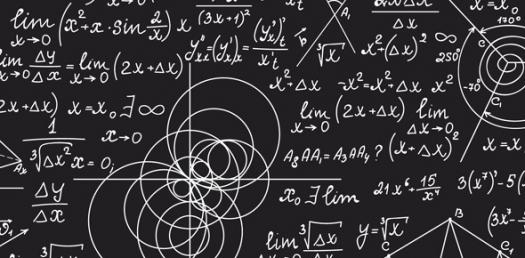# Math Challenge Quizzy Poo

Approved & Edited by ProProfs Editorial Team
At ProProfs Quizzes, our dedicated in-house team of experts takes pride in their work. With a sharp eye for detail, they meticulously review each quiz. This ensures that every quiz, taken by over 100 million users, meets our standards of accuracy, clarity, and engagement.
| Written by Lisabellclay
L
Lisabellclay
Community Contributor
Quizzes Created: 2 | Total Attempts: 771
Questions: 10 | Attempts: 217SettingsChallenge all of the math skills you have learned so far this year! Be sure to show off your score.

• 1.

### Which of the following is the greatest common factor of 6 and 10?

• A.

2

• B.

10

• C.

30

• D.

60

A. 2
Explanation
The greatest common factor (GCF) of two numbers is the largest number that divides both of them without leaving a remainder. In this case, the factors of 6 are 1, 2, 3, and 6, while the factors of 10 are 1, 2, 5, and 10. The common factors are 1 and 2, but the greatest common factor is 2 because it is the largest number that divides both 6 and 10 without leaving a remainder.

Rate this question:

• 2.

### Which are multiples of both 4 and 6?

• A.

18 and 20

• B.

20 and 24

• C.

12 and 24

• D.

18 and 20

C. 12 and 24
Explanation
The numbers 12 and 24 are multiples of both 4 and 6. This is because both 4 and 6 are factors of these numbers. For example, 12 can be divided evenly by both 4 (12/4=3) and 6 (12/6=2). Similarly, 24 can be divided evenly by both 4 (24/4=6) and 6 (24/6=4). Therefore, 12 and 24 are the correct answer.

Rate this question:

• 3.

### Which is equivalent to 3/20?

• A.

3%

• B.

5%

• C.

15%

• D.

20%

C. 15%
Explanation
The fraction 3/20 can be converted to a percentage by dividing the numerator (3) by the denominator (20) and multiplying by 100. This gives us 15%. Therefore, the option that is equivalent to 3/20 is 15%.

Rate this question:

• 4.

### Carl needs 2 and 2/3 cups of flour to make a cake.  he only has 3/8 cup of flour.  How much more flour does he need to make the cake?

• A.

2 and 1/24

• B.

2 and 1/5

• C.

2 and 7/24

• D.

2 and 5/11

C. 2 and 7/24
Explanation
Carl needs a total of 2 and 2/3 cups of flour to make a cake. He already has 3/8 cup of flour. To find out how much more flour he needs, we can subtract the amount he already has from the total amount needed. When we subtract 3/8 from 2 and 2/3, we get 2 and 7/24. Therefore, Carl needs 2 and 7/24 cups more flour to make the cake.

Rate this question:

• 5.

### 3.2 ÷ 0.2 =

• A.

2

• B.

16

• C.

64

• D.

160

B. 16
Explanation
When dividing 3.2 by 0.2, we can think of it as finding out how many times 0.2 can fit into 3.2. By dividing 3.2 by 0.2, we get the result of 16. Therefore, the correct answer is 16.

Rate this question:

• 6.

### Karla earns \$8.50 per hour at her part-time job.  Last wee she worked 18 hours.  What is the total amount of money she earned?

• A.

\$40.80

• B.

\$153.00

• C.

\$272.00

• D.

\$312.80

B. \$153.00
Explanation
Karla earns \$8.50 per hour at her part-time job and worked for 18 hours last week. To find the total amount of money she earned, we multiply her hourly rate (\$8.50) by the number of hours she worked (18). This gives us \$153.00. Therefore, the correct answer is \$153.00.

Rate this question:

• 7.

### John completed 60% of a running race before twisting his ankle.  What fraction of the race had he finished before getting hurt?

• A.

1/3

• B.

1/2

• C.

3/5

C. 3/5
Explanation
John completed 60% of the running race before twisting his ankle. This means that he finished more than half of the race but less than two-thirds of it. The only option that falls within this range is 3/5. Therefore, the fraction of the race that John had finished before getting hurt is 3/5.

Rate this question:

• 8.

### Mark has 7/8 of his birthday cake left.  He wants to give it to 5 people in equal pieces.  What fraction of the cake will each friend get to eat?

• A.

5/24

• B.

4 and 3/8

• C.

7/40

C. 7/40
Explanation
Mark has 7/8 of his birthday cake left. He wants to give it to 5 people in equal pieces. To find out what fraction of the cake each friend will get to eat, we need to divide the amount of cake Mark has by the number of people. Dividing 7/8 by 5 gives us 7/40. Therefore, each friend will get to eat 7/40 of the cake.

Rate this question:

• 9.

### What is the algebraic expression for the sentence below?                                   15 less than a number

• A.

15-n

• B.

N-15

• C.

15+n

B. N-15
Explanation
The algebraic expression for "15 less than a number" is n-15.

Rate this question:

• 10.

### What are the coefficients in the algebraic expression below?                     5s - 6 + 2 - 9c

• A.

5s, 6, 2, and 9c

• B.

5 and 9

• C.

S and c

• D.

6 and 2Back to top